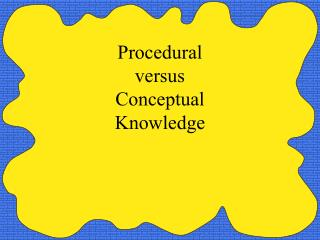DownloadDownload PresentationProcedural versus Conceptual Knowledge

# Procedural versus Conceptual Knowledge

Télécharger la présentation## Procedural versus Conceptual Knowledge

- - - - - - - - - - - - - - - - - - - - - - - - - - - E N D - - - - - - - - - - - - - - - - - - - - - - - - - - -
##### Presentation Transcript

1. Procedural versus Conceptual Knowledge

2. Invert and multiply.This is ProceduralKnowledge.Knowledge about an algorithm, that is a procedure to compute an answer.

3. A story about Procedural knowledge. Told by researchers About 5th Graders in North Carolina Who had learned well how to invert and multiply

4. After lunch on a day that the cafeteria served pizza, the researchers posed a question. What is one-half of a pizza divided by one-fourth of a pizza?

5. The students inverted and multiplied and said that the answer was 2. “Two what?” the researchers asked. After some thought and discussion, the student responded, “2 pizzas”.

6. What do you mean, 2 pizzas. Drawings were made of two whole pizzas. How can that be? We divided 1/2 of a pizza by 1/4 of a pizza. Where did all that pizza come from. After much thought the students responded, “ We don’t know where the pizza came from, but we know the answer is 2.

7. The students’ misunderstanding occurred because they only had the Procedural knowledge--Invert and Multiple. They did not understand what they were doing at the conceptual level.

8. They have not connected the algorithm with real/concrete/pictorial situations. They lacked the “conceptual knowledge” to understand.

9. Fraction Circles 1/4 1/2

10. 1/4 1/2

11. Benefit of Conceptual Knowledge You UNDERSTAND the math that you are doing. Students should always have conceptual knowledge before they learn an algorithm.

12. Part 2 Another Story Sometimes I reminisce about my high school days. What comes to mind is Geometry Class and a formula I learned.

13. The Formula: (N-2) * 180o = the sum of the interior angles of a polygon. An Example: (5-2) * 180o = 3 *180o = 540o

14. I remember the teacher showed me this formula. I remember it was in our textbook. I remember that I remembered it for a test. I remember that I forgot it soon afterwards. I forgot it because I never understood it, I only memorized it.

15. How Could I Have Learned It Better? • Explore • Investigate • Conjecture • Discover • Construct • Describe

16. Two opposing examples of how to teach N-2.